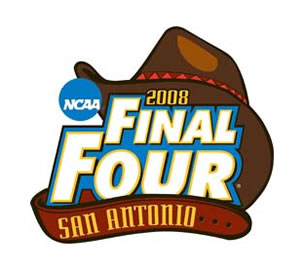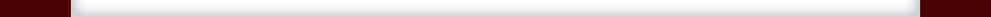# Steve Cotler## NCAA Final Four MathBy missing their last shot, tenth-seeded Davidson lost to top-seeded Kansas 59-57…and for the first time in NCAA tournament history, all four #1 seeds made it to the Final Four.

What are the odds of that happening?

My assumptions are arbitrary—I admit that—but here’s the math.

Assume that the #1 seed is is X percent better than the #2 seed, which is X percent better than the #3 seed, and so forth. And assume that if a lower seed upsets a higher seed, then the winner is assumed to be as good at the team it has beaten.

If X = 1%, then each #1 seed has a 10.4% of winning its bracket, yielding a 0.01% chance that all four #1 seeds win, or one chance out of 8700.

If X = 5%, then each #1 seed has a 11.1% of winning its bracket, yielding a 0.02% chance that all four #1 seeds win, or one chance out of 6600.

If X = 10%, then the numbers are 17.1% and 0.09%…1 out of 1200.

If X = 20%—in my opinion, an overlarge number—then the chances are 29.3% and 0.74%…1 out of 134.

Schwertman, et al. in Anthology of Statistics in Sports, employs a simpler formula: if a #X seed plays #Y seed, then assume that X’s probability of winning equals Y divided by the sum of X + Y. So when a #1 seed plays a #16, the top-seeded team has a 16/17 chance of winning. Using this rationale, the chances are 17.2% and 0.09%…1 out of 1150.

So, with only 30 years of seeded NCAA Final Fours as a universe, the actual result is truly a statistical rarity.

# * * * * *

Updated 4/8/08 after Kansas won it all. Details of my probability calculations available upon request.